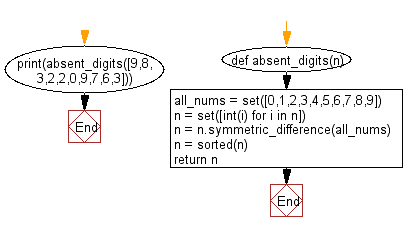﻿ Python: Find the digits which are absent in a given mobile number - w3resource# Python: Find the digits which are absent in a given mobile number

## Python Basic - 1: Exercise-25 with Solution

Write a Python program to find the digits which are absent in a given mobile number.

Sample Solution:

Python Code:

``````def absent_digits(n):
all_nums = set([0,1,2,3,4,5,6,7,8,9])
n = set([int(i) for i in n])
n = n.symmetric_difference(all_nums)
n = sorted(n)
return n
print(absent_digits([9,8,3,2,2,0,9,7,6,3]))
``````

Sample Output:

```[1, 4, 5]
```

Pictorial Presentation:Flowchart:## Visualize Python code execution:

The following tool visualize what the computer is doing step-by-step as it executes the said program:

Python Code Editor:

Have another way to solve this solution? Contribute your code (and comments) through Disqus.

What is the difficulty level of this exercise?

Test your Python skills with w3resource's quiz

﻿

## Python: Tips of the Day

Checks if the given number falls within the given range.

Example:

```def tips_range(n, start, end = 0):
return start <= n <= end if end >= start else end <= n <= start
print(tips_range(2, 4, 6))
print(tips_range(4, 8))
print(tips_range(1, 3, 5))
print(tips_range(1, 3))
```

Output:

```False
True
False
True
```